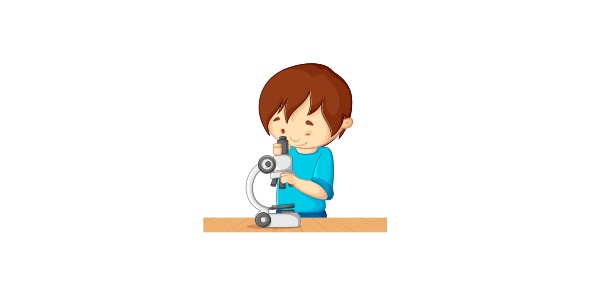26 Questions | Attempts: 727
ShareSettingsSelect the most appropriate answer from the choices given.

• 1.
In a flowchart, which basic operation is a rectangle used to represent?
• A.

Decision

• B.

Input/Output

• C.

Process/Assignment

• D.

Storage

• 2.
Which of the following is not a significant part of a simple problem?
• A.

Input

• B.

Processing

• C.

Output

• D.

Solution

• 3.
During problem decomposition, expected results are documented in the _____________ column in the defining diagram.
• A.

Input

• B.

Processing

• C.

Output

• D.

Storage

• 4.
Developing and representing algorithms are included in the _______ step in the problem solving process.
• A.

First

• B.

Third

• C.

Fourth

• D.

Last

• 5.
ALL of the following are true about variables and constants EXCEPT that _______
• A.

The have names called identifiers.

• B.

Their names refer to an area of memory.

• C.

Their names are created by the programmer.

• D.

They can be used interchangeably.

• 6.
Which of the following is an incorrect variable name?
• A.

1stchoice

• B.

Firstchoice

• C.

FirstChoice

• D.

FIRSTCHOCIE

• 7.
A ________ is a named location in memory, the value of which remains fixed.
• A.

Constant

• B.

Literal

• C.

Half-constant

• D.

Variable

• 8.
Which data type is MOST appropriate for storing a value representing the weight of a new born child?
• A.

Character

• B.

Integer

• C.

Real

• D.

String

• 9.
What is the output of the following algorithms, if a is 5 and b is 9? Read a, b a= a+b b= a/2 Print a, b
• A.

5, 9

• B.

14, 7

• C.

14, 9

• D.

5, 7

• 10.
Which condition will determine if n is of a higher value than 10?
• A.

N >= 10

• B.

N > 10

• C.

N

• D.

N < 10

• 11.
Consider the following: Five numbers are to be input (under the control of a loop). The biggest number must be determined and output. How many columns are necessary for the required trace table?
• A.

6

• B.

5

• C.

3

• D.

2

• 12.
The number of ________ in an algorithm determines the number of rows in a trace table.
• A.

Constants

• B.

Calculations

• C.

Passes

• D.

Variables

• 13.
Pseudocode is a __________ representation of algorithms
• A.

Graphical

• B.

Textual

• C.

Verbal

• D.

Visual

• 14.
Which statement is used to determine if the value of x is the same or less than the value of y?
• A.

If x < y

• B.

If x

• C.

If x > y

• D.

If x >= y

• 15.
A _________ is a named location in memory, the value of which changes during program execution.
• A.

Constant

• B.

Literal

• C.

Sentinel

• D.

Variable

• 16.
An algorithm specifies the flow of control from one ________ to another.
• A.

Structure

• B.

Value

• C.

Process

• D.

Variable

• 17.
Which algorithm will accept, add and then calculate and output the mean of TWO numbers?
• A.

Sum = n1 + n2 read n1, n2 write sum write avg

• B.

Sum = n1 + n2 read n1, n2 sum = n1 + n2 write avg

• C.

Read n1, n2 sum = n1 + n2 avg = sum/2 write avg

• D.

Read n1, n2 sum= n1 + n2/2 avg = sum/2

• 18.
In flowcharting, which basic operation is a diamond used to represent?
• A.

Decision

• B.

Input/Output

• C.

Process/assignment

• D.

Storage

• 19.
Which statement is used to determine and output the bigger of two unequal numbers?
• A.

IF n1 > n2 THEN print n2 else print n1

• B.

IF n1 < n2 THEN print n2 ELSE print n1

• C.

IF n1 > n2 THEN print n2 ELSE print n1

• D.

IF n1 > n2 THEN print n1 ELSE print n2

• 20.
Which of the following descriptions are characteristics of an algorithm? i. Precise ii. Ambiguous iii. Terminable iv. Unambiguous
• A.

I and ii

• B.

I, ii and iii

• C.

I, ii and iv

• D.

I, iii and iv

• 21.
State the correct DATA TYPE for the example provided. 45
• 22.
State the correct DATA TYPE for the example provided. 3.14
• 23.
State the correct DATA TYPE for the example provided. A
• 24.
State the correct DATA TYPE for the example provided. Maria
• 25.
State the correct DATA TYPE for the example provided. Cloudy = true Sunny = false

## Related TopicsBack to top
×

Wait!
Here's an interesting quiz for you.